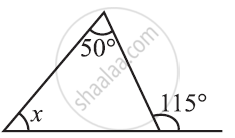Share

# Find the Value of the Unknown Interior Angle X In the Following Figure. - 1 - CBSE Class 7 - Mathematics

ConceptExterior Angle of a Triangle and Its Property

#### Question

Find the value of the unknown interior angle x in the following figure.#### Solution

x + 50° = 115° (Exterior angle theorem)

x = 115° − 50° = 65°

Is there an error in this question or solution?

#### APPEARS IN

NCERT Solution for Mathematics for Class 7 (2018 to Current)
Chapter 6: The Triangle and its Properties
Ex. 6.20 | Q: 2.1 | Page no. 119
Solution Find the Value of the Unknown Interior Angle X In the Following Figure. - 1 Concept: Exterior Angle of a Triangle and Its Property.
S